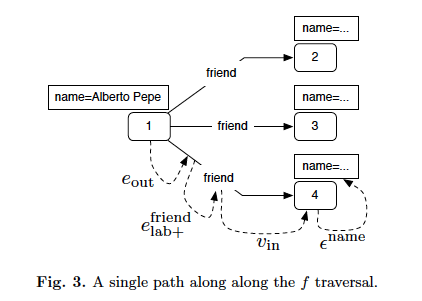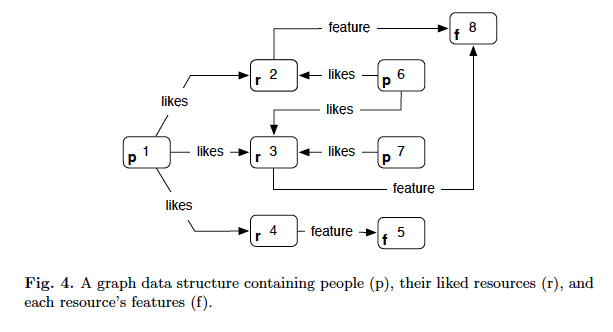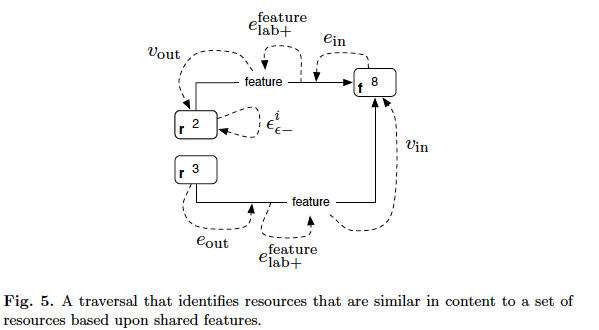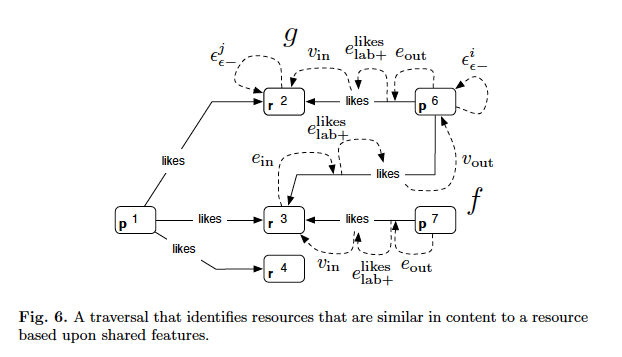# Data Infrastructure - Graph Traversal Pattern II

### Graph Traversals

A traversal means visting elements (vertices and edges) in a graph in some algorithmic fashion. This subsection will introduce a functional flowbased approach to traversing property graphs and how different types of traversals over different types of graph datasets support different types of problem-solving.

The most primitive, read-based operation on a graph is a single step traversal from element $i$ to element $j$, where $i,j \in (V \cup E)$. For example, “Find the outgoing edges from this vertex”,”Find the vertex that is at the end of this edge”. Single step operations expose explicit adjacencies in the graph. Following list itemizes the various types of single step traversals.

$e_{out}$ : traverse to the outgoing edges of the vertices
$e_{in}$ :traverse to the incoming edges to the vertices
$v_{out}$ : traverse to the outgoing (or say tail) vertices of the edges
$v_{in}$ : traverse the incoming(or say head) vertices of the edges
$\epsilon$ : get the element property values for given key

When edges are labeled and elements have properties, it is desirable to constrain the traversal to edges of a particular label or elements with particular label or elements with particular properties. These operations are known as filters and are abstractly defined as following:

$e_{lab+}$ allow all edges with label
$e_{lab-}$ filter all edges with label
$\epsilon_{p+}$ allow all elements with the property
$\epsilon_{p-}$ filter all elements with the property
$\epsilon_{\epsilon +}$ allow all elements that are the provided element
$\epsilon_{\epsilon -}$ filter all elements that are the provided element

A simple example is traversing to the names of Alberto Pepe’s friends of last post. If $i$ is the vertex representing Alberto Pepe.

$f(i) = \epsilon(v_{int}(e_{lab+}(e_{out}(i),friend)), name)$

then $f(i)$ will return the names of Alberto Pepe’s friends. By function currying and composition, we can rewrite the above definition.

$f(i) = (\epsilon^{name} \cdot v_{in} \cdot e^{friend}_{lab+} \cdot e_{out})(i)$### Traversal Pattern of Recommendation

Like I mentioned in last post, currently every giant tech company has some sort of graph data. In this stage, every company want to do some sort of recommendation to increase user engagement.So let us focus on traversal pattern of recommendation. Following is how the graph looks like.### Content-Based Recommendation

In order to identify resources that are similar in features to a resource, traverse to all resources that share the same features. Here is the traversal pattern function definition. Let $i$ be the resource

$f(i) = (\epsilon^{i}_{\epsilon -} \cdot v_{out} \cdot e^{feature}_{lab+} \cdot e_{in} \cdot v_{in} \cdot e^{feature}_{lab+} \cdot e_{out}) (i)$

Following figure describe the processing.There is one important extension to this problem : “Given what person $i$ likes, what other resources have similar features?” We just need to define an additinal function $g$ of person $i$

$g(i) = (v_{in} \cdot e^{likes}_{lab+} \cdot e_{out}) (i)$

and then our problem can be solved by $f \dot g$

### Collaborative Filtering-Based Recommendation

In the world of recommendation, there is another recommendation called “collaborative filtering based recommendation”. With collaborative filtering, the objective is to identify a set of resources that have a high probability of being liked by a person based upon identifying other people in the system that enjoy similar likes. For exampl, if person $a$ and person $b$ share 90% of their liked resources in common, then the remaining 10% they don’t share in common are candidates for recommendation. Solving the problem of collaborative filtering using graph traversals can be accomplished 2 components.

$f(i) = (\epsilon^{i}_{\epsilon -} \cdot v_{out} \cdot e^{like}_{lab+} \cdot e_{in} \cdot v_{in} \cdot e^{like}_{lab+} \cdot e_{out}) (i)$

Function $f$ traverses to all those people vertices that like the same resources as person vertex $i$ and who themselves are not vertex $i$.

$g(j) = (v_{in} \cdot e^{like}_{lab+} \cdot e_{out}) (j)$

Fucntion $g$ traverses to all the resources liked by vertex $j$. In composition, $(g \cdot f)(i)$ determines all those resources that are liked by those people that have similar tastes to vertex $i$. If person $j$ likes 10 resources in common with person $i$, then the resources that person likes will be returned at least 10 times by $g \cdot f$ (perhaps more if a path exists to those resources from another person vertex as well)### Conclusion

With the graph traversal pattern, there exists a single graph data structure that can be traversed in different ways to expose different types of recommendations|generally, different types of relationships between vertices.Being able to mix and match the types of traversals executed alters the semantics of the final rankings and conveniently allows for hybrid recommendation algorithms to emerge.